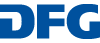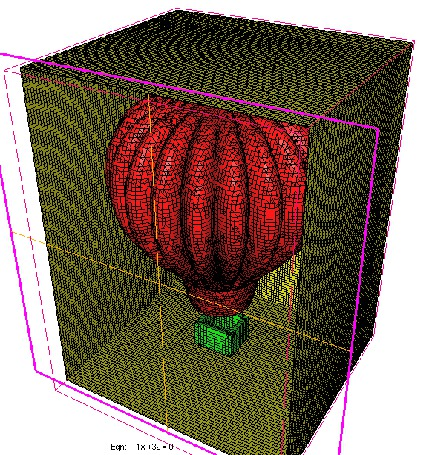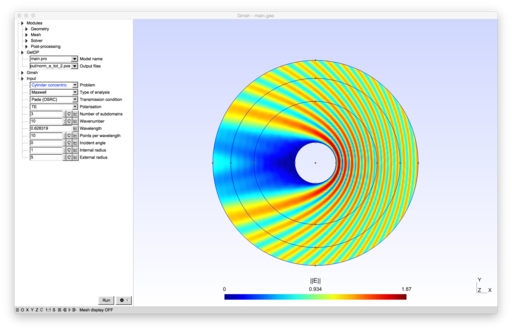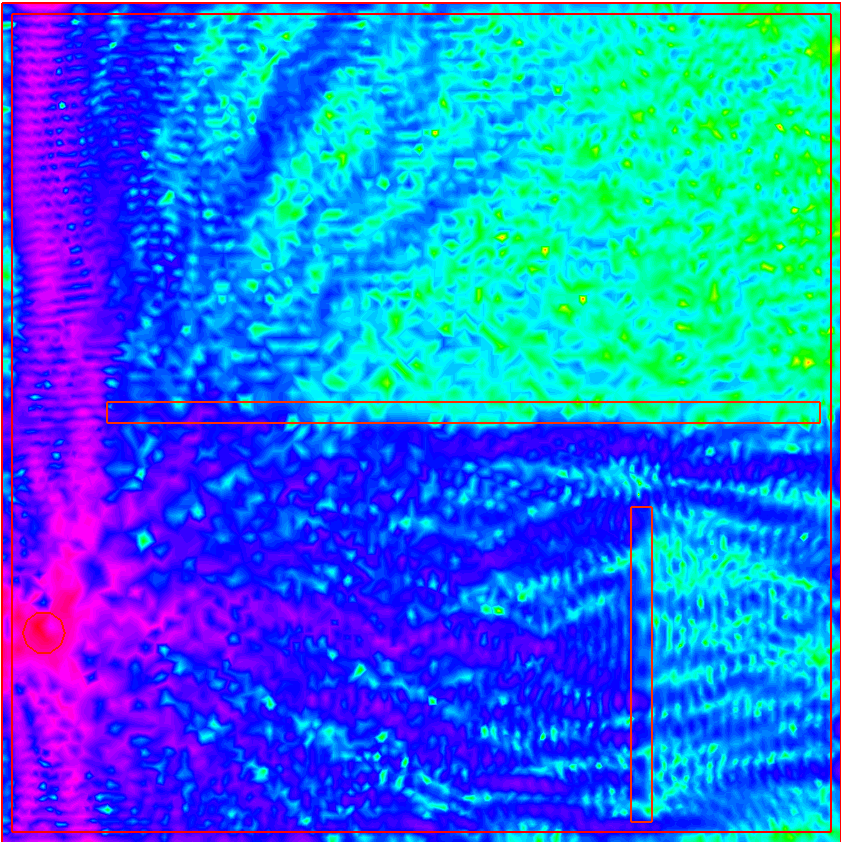You are here:   HomeLight Scattering SoftwareFinite Elements Codes 28 | 09 | 2023
Related / External
Acknowledgement
We acknowledge support of this project by Deutsche Forschungsgemeinschaft (DFG) within the special program "Themenorientierte Informationsnetzwerke".The page is hosted by the University of Bremen.### Gyptis

Gyptis is a package to solve Maxwell’s equations with the finite element method. It is in early stage and currently being actively developed, so features might come and go.### XLiFE++

XLiFE++ is an FEM-BEM C++ code developed by P.O.e.m.s. laboratory and I.R.M.A.R. laboratory, that can solve 1D / 2D / 3D, scalar / vector, transient / stationnary / harmonic problems.

It includes Boundary Elements methods for Maxwell equations.### Montjoie

Montjoie is a high-order finite element C++ framework, to solve time-domain or time-harmonic linear partial differential equations. There are several finite element : nodal finite elements, discontinuous Galerkin finite elements, edge finite elements.### Onelab Electromagnetic Scattering

Onelab model for solving various 3D electromagnetic scattering problems on an isolated object. Onelab (Open Numerical Engineering LABoratory) is an open-source, lightweight interface to finite element software. The T-matrix of an isolated scatterer of arbitrary shape can be computed.### ElectromagneticScattering

A Onelab model for 3D scattering problems in nanophotonics. The T-matrix of an isolated scatterer of arbitrary shape can be computed.GetDDM combines GetDP and Gmsh to solve large scale finite element problems using optimized Schwarz domain decomposition methods.### FreeFEM

FreeFEM is a partial differential equation solver for non-linear multi-physics systems in 2D and 3D. Problems involving partial differential equations from several branches of physics, such as fluid-structure interactions, require interpolations of data on several meshes and their manipulation within one program. FreeFEM includes a fast interpolation algorithm and a language for the manipulation of data on multiple meshes.JFEM3D

JEM3D ist an FEM solver by Jeffrey M. McMahon which solves the vector wave equation for the electric or magnetic field directly.### ERMES

ERMES (Electric Regularized Maxwell Equations with Singularities) is a finite element code in frequency domain which implements in C++ a simplified version of the weighted regularized Maxwell equation method.Librairie Eléments Finis Mélina: Documentation, TéléchargementSpecial